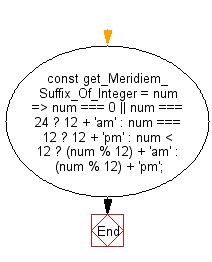# JavaScript : Convert an integer to a suffixed string, adding am or pm based on its value

## JavaScript fundamental (ES6 Syntax): Exercise-50 with Solution

Write a JavaScript program to convert an integer to a suffixed string, adding am or pm based on its value.

• Use the modulo operator (%) and conditional checks to transform an integer to a stringified 12-hour format with meridiem suffix.

Sample Solution:

JavaScript Code:

``````//#Source https://bit.ly/2neWfJ2
const get_Meridiem_Suffix_Of_Integer = num =>
num === 0 || num === 24
? 12 + 'am'
: num === 12
? 12 + 'pm'
: num < 12
? (num % 12) + 'am'
: (num % 12) + 'pm';

console.log(get_Meridiem_Suffix_Of_Integer(0));
console.log(get_Meridiem_Suffix_Of_Integer(11));
console.log(get_Meridiem_Suffix_Of_Integer(13));
console.log(get_Meridiem_Suffix_Of_Integer(25));
```
```

Sample Output:

```12am
11am
1pm
1pm
```

Pictorial Presentation:Flowchart:Live Demo:

See the Pen javascript-basic-exercise-1-49 by w3resource (@w3resource) on CodePen.

Improve this sample solution and post your code through Disqus

What is the difficulty level of this exercise?

Test your Programming skills with w3resource's quiz.

﻿

## JavaScript: Tips of the Day

Chunks an array into n smaller arrays

Example:

```const tips_chunkIntoN = (arr, n) => {
const size = Math.ceil(arr.length / n);
return Array.from({ length: n }, (v, i) =>
arr.slice(i * size, i * size + size)
);
}
console.log(tips_chunkIntoN([1, 2, 3, 4, 5, 6, 7,8], 4));
```

Output:

```[[1,2],[3,4],[5,6],[7,8]]
```